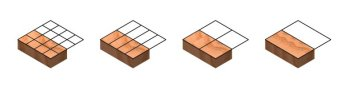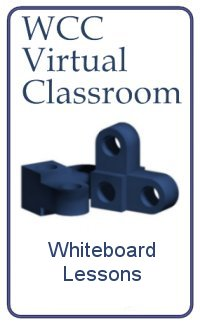Computer-Aided Design Engineering Programming Architectural Design Mechanical Design Civil Design Energy Management Electrical Design

Network
Engineer

Project
Management
 Table of ContentsWhen working with fractions, we need to be able to convert the value to a proportional number so we can add or subtract them. As shown in the image above, by dividing both the numerator and the denominator by two reduces 8/16 to 4/8, then 4/8 to 2/4, and finally 2/4 to 1/2. Therefore, we now know that 8/16 = 4/8 = 2/4 = 1/2. We could have easily gone in the other direction, multiplying both the numerator and the denominator by two never completing the task since both parts of the fraction would go to infinity. At World Class CAD, we use computer applications to solve every type of challenge. Working with fractions is just one. Title Page Ch.1: Working with Fractions Appendix A: Adding Fractions Appendix B: Subtracting Fractions Appendix C: Multiplying Fractions Appendix D: Dividing Fractions Prime Numbers Exercise Measuring Lab Assignments Tools for Successful Math Training Reference Multiplication Table Unit Conversion Chart Fraction to Decimal Conversion Chart Using a English Ruler or Metric Scale Metric Conversion Chart Exercises, Quizzes and Tests Week 1 Math Warmup Week 1 Fractions Pretest Week 1 Measuring Lab Week 1 Measuring Lab Exercise Week 2 Review Week 2 Test Week 3 Solve for N - Exercise 1 Week 3 Solve for N - Exercise 2 Week 3 Solve for N - Exercise 3 Week 3 Solve for N - Exercise 4 Week 3 Solve for N - Pop Quiz 1 Week 3 Solve for N - Pop Quiz 2 Week 3 Fraction Assessment 2 Week 3 Fraction Exercise Week 3 Math Lab 1 Week 3 Math Lab 2 Week 4 Percentages Week 4 Solve for N and Percent Exercise 1 Week 4 Solve for N and Percent Exercise 2 Week 4 Metric and Percentage Exercise Week 4 Conversion Exercise 1 Week 4 Conversion Exercise 2 Week 4 Fraction Exercise Week 4 Review Week 4 Test Week 5 Metric Conversion Reference Week 5 Conversion Samples Week 5 Conversion Exercise Week 5 Conversion Exercise - 4 page Week 5 Conversion Quiz 1 Week 5 Conversion Quiz 2 Week 5 Conversion Quiz 3 Week 5 Converting Celsius and Fahrenheit Week 5 Real World Conversion Aps Week 5 Metric Conversion Review 1 Online Quiz: Metric Conversion Quiz 1 Week 5 Metric Conversion Review 2 Online Quiz: Metric Conversion Quiz 2 Week 5 Metric Conversion Review 3 Online Quiz: Metric Conversion Quiz 3 Week 5 Metric Conversion Review 4 Online Quiz: Metric Conversion Quiz 4 Week 5 Metric Conversion Review 5 Week 6 Exercise - Working with Angles Week 6 Scientific Notation Worksheet Week 6 Scientific Notation Activity Week 6 Basic Scientific Notation Quiz Week 6 Multiply & Divide Scientific Not.Quiz Week 6 Multiply & Divide Scientific Not. Quiz 2 Week 6 Scientific Notation Applications Week 6 Scientific Notation Quiz Week 6 Review Week 7 Graphing Lab